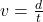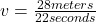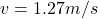## Question 8 of 10 It takes a person 22 seconds to swim in a straight line from the south end of a pool to the north end of the po

Question

Question 8 of 10
It takes a person 22 seconds to swim in a straight line from the south end of
a pool to the north end of the pool, a distance of 28 meters. What is the
swimmer’s velocity?
A. 1.3 m/s south
B. 1.3 m/s north
C. 0.8 m/s south
D. 0.8 m/s north

in progress 0
3 months 2021-07-31T17:02:45+00:00 1 Answers 3 views 0

1. The correct answer is B. 1.3 m/s north

Explanation:

Velocity is a factor that describes how fast or slow the motion of a body occurs and its direction. Moreover, this can be calculated by dividing the total displacement into the time of movement, and the final result is expressed in units such as meters per second followed by the direction, for example, 152 m/s south. The process to calculate the velocity of the swimmer is shown below.This means the velocity of the swimmer is 1.27 m/s, which can be rounded as 1.3 m/s. Additionally, if the direction is considered it would be 1.3 m/s north because the swimmer went from the south of the pool to its north.